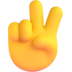# Normalized vector from an entity's angles.

How can I do so?

You can get three vectors:

[lua]local forward = angle:Forward();
local right = angle:Right();
local up = angle:Up();[/lua]

I know, but they all return an Angle object, I need to get the normal of all the 3 directions(pitch, yaw, roll).

They do return normalized vectors though.

But they return a vector for each direction.

Anyway to put my question bluntly:

[lua]
include(“shared.lua”)

local html = vgui.Create(“HTML”)
html:SetPos(ScrH(), ScrW())
html:SetSize(256, 256)
html:SetHTML([[
<html>
</body>
This is a test
</body>
</html>
]])

function ENT:Draw()
self:DrawModel()
self:DrawPanel()
end

function ENT:DrawPanel( )
if LocalPlayer():GetPos():Distance(self:GetPos()) < 700 then
if html:GetHTMLMaterial() then
cam.Start3D2D( LocalPlayer():EyePos(), LocalPlayer():EyeAngles())
render.SetMaterial(html:GetHTMLMaterial())
render.DrawQuadEasy( self:GetPos(),
Vector(/i need to put the normals here/),
128, 128,
Color( 255, 255, 255, 255 ),
180 )
cam.End3D2D()
end
end
end
[/lua]

The vector I need is the direction of the plane.

LocalPlayer():EyeAngles():Forward()

If you want the direction the entity is pointing in, use Forward.

That doesn’t work.

You’re using render.DrawQuadEasy wrong. You shouldn’t use 3D2D for that.

-1 * LocalPlayer():EyeAngles():Forward()

If the quad you are drawing is facing the same way as the player, it will have its back turned to you, and basically you will see nothing. It has to face the opposite way, so basically it has to face you.

[editline]7th November 2010[/editline]

That too.I don’t want the quad to face to me, I want the quad to have the same rotation of the entity “placeholder” (the code I posted above is from an entity)